### Home > INT3 > Chapter 12 > Lesson 12.1.1 > Problem12-9

12-9.
1. Some of the following rational expressions have common denominators and some do not. Add or subtract the expressions and, if possible, simplify. Homework Help ✎

1.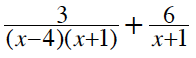2.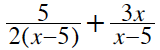3.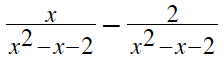4.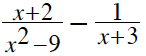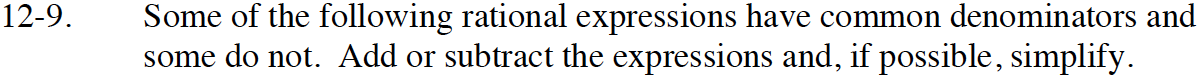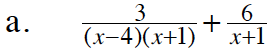$\frac{3}{(x-4)(x+1)}+\frac{6}{x+1}\cdot \frac{x-4}{x-4}$

$\frac{3+6(x-4)}{(x-4)(x+1)}$

$\frac{6x-21}{(x-4)(x+1)}$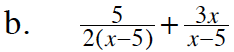See part (a).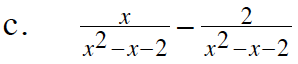Try factoring after subtracting.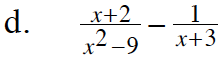Factor to find the common denominator, then use the strategy from part (a) to simplify.

$\frac{5}{x^2-9}$

$\text{If your answer was }-\frac{1}{x²-9},$

check the step where you subtracted.

−(x − 3) = x + 3